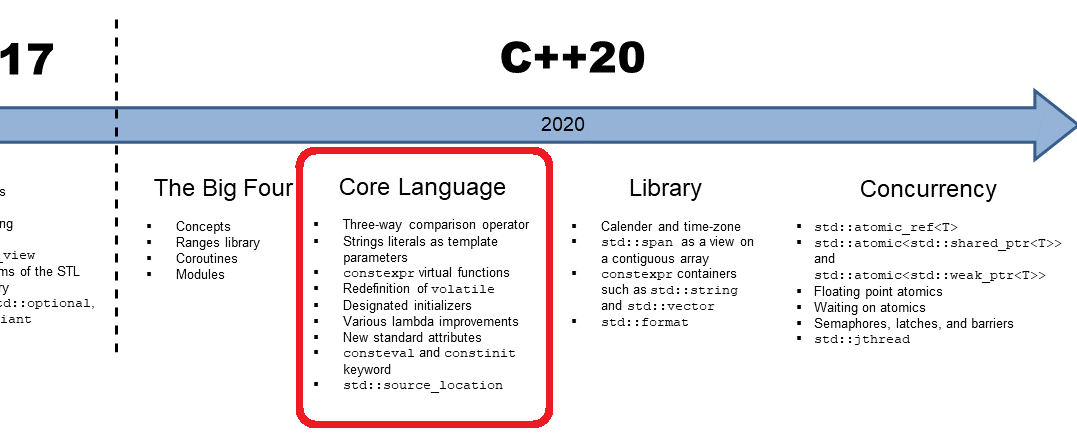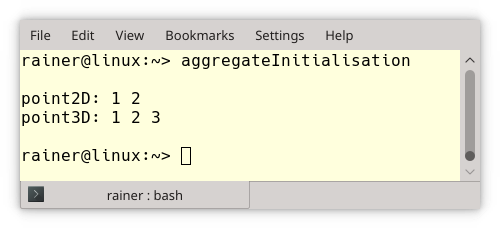# C++20：核心语言## 核心语言### 三元比较运算符 <=>

#include <compare>struct MyInt {  int value;  MyInt(int value): value{value} { }  auto operator<=>(const MyInt&) const = default;};

struct Basics {  int i;  char c;  float f;  double d;  auto operator<=>(const Basics&) const = default;}; struct Arrays {  int ai;  char ac;  float af;  double ad;  auto operator<=>(const Arrays&) const = default;}; struct Bases : Basics, Arrays {  auto operator<=>(const Bases&) const = default;}; int main() {  constexpr Bases a = { { 0, 'c', 1.f, 1. },                        { { 1 }, { 'a', 'b' }, { 1.f, 2.f, 3.f }, { { 1., 2. }, { 3., 4. } } } };  constexpr Bases b = { { 0, 'c', 1.f, 1. },                        { { 1 }, { 'a', 'b' }, { 1.f, 2.f, 3.f }, { { 1., 2. }, { 3., 4. } } } };  static_assert(a == b);  static_assert(!(a != b));  static_assert(!(a < b));  static_assert(a <= b);  static_assert(!(a > b));  static_assert(a >= b);}

### 字符串文本作为模版参数

template<std::basic_fixed_string T>class Foo {    static constexpr char const* Name = T;public:    void hello() const;}; int main() {    Foo<"Hello!"> foo;    foo.hello();}

### 指定初始化值

// aggregateInitialisation.cpp #include <iostream> struct Point2D{    int x;    int y;}; class Point3D{public:    int x;    int y;    int z;}; int main(){        std::cout << std::endl;        Point2D point2D {1, 2};    Point3D point3D {1, 2, 3};     std::cout << "point2D: " << point2D.x << " " << point2D.y << std::endl;    std::cout << "point3D: " << point3D.x << " " << point3D.y << " " << point3D.z << std::endl;        std::cout << std::endl; }// designatedInitializer.cpp #include <iostream> struct Point2D{    int x;    int y;}; class Point3D{public:    int x;    int y;    int z;}; int main(){        std::cout << std::endl;        Point2D point2D {.x = 1, .y = 2};    // Point2D point2d {.y = 2, .x = 1};         // (1) error    Point3D point3D {.x = 1, .y = 2, .z = 2};       // Point3D point3D {.x = 1, .z = 2}          // (2)  {1, 0, 2}         std::cout << "point2D: " << point2D.x << " " << point2D.y << std::endl;    std::cout << "point3D: " << point3D.x << " " << point3D.y << " " << point3D.z << std::endl;        std::cout << std::endl; }

Point2D 和 Point3D 实例的参数是被显式声明的。该程序的输出与程序 aggregateInitialisation.cpp 的输出相同。注释掉的行（1）和（2）非常有趣。行（1）将会产生错误，因为指定元素的顺序与其声明顺序不匹配。 y 的指定值在行（2）中是缺失的。在这种情况下，y 将被初始化为 0，类似于使用带括号的初始化列表 {1、0、3} 对其进行初始化。

### 各种 Lambda 的改进

• ** 允许 [=, this] 作为 Lambda 捕获器，并弃用隐式 this 捕获器 [=] **

struct Lambda {    auto foo() {        return [=] { std::cout << s << std::endl; };    }     std::string s;}; struct LambdaCpp20 {    auto foo() {        return [=, this] { std::cout << s << std::endl; };    }     std::string s;};

• 模版 Lambda

template <typename T>T operator(T x) const {    return x;}

auto foo = []<typename T>(std::vector<T> const& vec) {         // do vector specific stuff    };

### 新属性：[[likely]] 和 [[unlikely]]

for(size_t i=0; i < v.size(); ++i){  if (unlikely(v[i] < 0)) sum -= sqrt(-v[i]);  else sum += sqrt(v[i]);}

### consteval 和 constinit 声明符

consteval int sqr(int n) {  return n*n;}constexpr int r = sqr(100);  // OK int x = 100;int r2 = sqr(x);             // Error

constinit 可以确保具有静态存储持续时间的变量在编译时初始化。静态存储持续时间意味着在程序开始时分配对象，在程序结束时释放对象。在命名空间作用域中声明的对象（全局对象）、声明为 static 或 extern 对象都具有静态存储持续时间。

### std::source_location

C++ 11 中有两个宏 LINE  和  FILE ，它们可用于在使用宏时获取信息。使用 C++ 20 ，source_location 类为我们提供了源代码的文件名、行号、列号和函数名等。下面是一个摘自 cppreference.com 的简短示例，它展示了第一种用法：

#include <iostream>#include <string_view>#include <source_location> void log(std::string_view message,         const std::source_location& location = std::source_location::current()){    std::cout << "info:"              << location.file_name() << ":"              << location.line() << " "              << message << '\n';} int main(){    log("Hello world!");  // info:main.cpp:15 Hello world!}

## 接下来的安排？

C++ 20: The Core Language

## 评论2019 年 11 月 12 日 11:35

2019 年 11 月 12 日 14:20

😄
2019 年 11 月 25 日 11:25